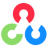Back to Adaptive Vision Studio website

# Structural Analysis and Shape Descriptors

Select a filter from the list below.

Icon Name Description ModulescvApproxPolyDP Approximates polygonal curve(s) with the specified precision. OpenCVcvArcLength Calculates a contour perimeter or a curve length. OpenCVcvBoundingRect Calculates the up-right bounding rectangle of a point set. OpenCVcvConnectedComponents Computes the connected components labeled image of boolean image. OpenCVcvContourArea Calculates the contour area. OpenCVcvConvexHull Finds the convex hull of a point set. OpenCVcvDrawContours Draws contour outlines or filled contours. OpenCVcvFindContours Finds the contours in a binary image. OpenCVcvFitEllipse Fits an ellipse around a set of 2D points. OpenCVcvFitLine Fits a line to a 2D or 3D point set. OpenCVcvHuMoments Calculates Hu moments of the image. OpenCVcvIsContourConvex Tests contour convexity. OpenCVcvMatchShapes_Images Compares two shapes. OpenCVcvMatchShapes_Polygon Compares two shapes. OpenCVcvMinAreaRect Finds a rotated rectangle of the minimum area enclosing the input 2D point set. OpenCVcvMinEnclosingCircle Finds the minimum area circle enclosing a 2D point set. OpenCVcvMinEnclosingTriangle Finds a rotated rectangle of the minimum area enclosing the input 2D point set. OpenCVcvMoments Calculates image moments. OpenCVcvPointPolygonTest Performs point-in-contour test. OpenCV